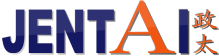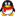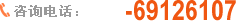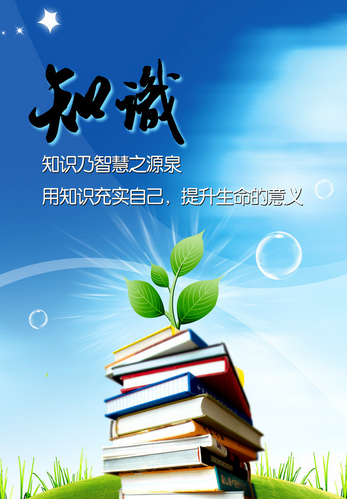### 专业热熔胶、502胶水、白乳胶制造商### 关于政太# 【政太纳米感悟：这才是人生的真谛！】

[点击量：1125][来源：政太纳米热熔胶www.oak-bond.com]

2014-12-16

Knowledge (知识)： K+N+O+W+L+E+D+G+E＝ 11+14+15+23+12+5+4+7+5=96%。

Workhard (努力工作）：W+O+R+K+H+A+R+D＝ 23+15+18+11+8+1+18+4 =98%。Luck（好运） L+U+C+K＝12+21+3+11=47%。

Love（爱情） L+O+V+E＝12+15+22+5=54%。

L+E+A+D+E+R+S+H+I+P=12+5+1+4+5+18+19+9+16=89%

ATTITUDE (心态) A+T+T+I+T+U+D+E＝1+20+20+9+20+21+4+5=100%。